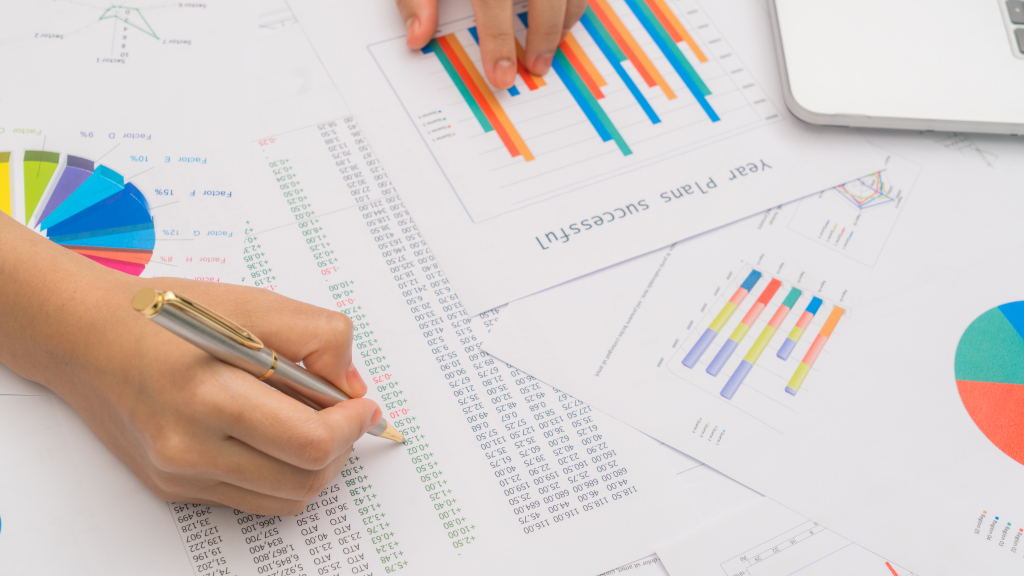# Ten days of statistics (8) - Correlationship## Covariance

This is a measure of how two random variables $X$ and $Y$ change together. Formally

$cov(X,Y) = \frac{1}{n}\sum_{i=1}^n (x_i - \bar{x}) \times (y_i - \bar{y})$

Where

• $\bar{x}$ is the mean of $x$
• $\bar{y}$ is the mean of $y$

## Pearson correlation coefficient

The Pearson correlation coefficient $\rho(X, Y)$ is given by:

$\rho(X,Y) = \frac{cov(X,Y)}{\sigma_X\sigma_Y}$

Where

• $\sigma_X$ is the standard deviation of $X$
• $\sigma_Y$ is the standard deviation of $Y$

## Spearman’s rank correlation coefficient

Given 2 random variables $X$ and $Y$ with the same sample size. Let $rank_X$ and $rank_Y$ denotes the ranks of each data point on $X$ and $Y$ respectively. Let $r_S$ is the Spearman’s rank correlation coefficient of $X$ and $Y$, which equal to the Pearson correlation coefficient of $rank_X$ and $rank_Y$

$r_S = \frac{cov(rank_X, rank_Y)}{\sigma_{rank_X}\sigma_{rank_Y}}$

If X and Y contains no duplicates

$r_S = \frac{cov(rank_X, rank_Y)}{\sigma^2}$

## Practice

Hackerrank has some exercises for you to test your knowledge:

Next lesson: Linear regression

That's all! Thank you for reading all the way here 😊

Made with love using Svelte, Three.js, TailwindCSS